Next: Ambipolar Diffusion Up: Ambipolar Diffusion Previous: Ambipolar Diffusion   Contents

## Ionization Rate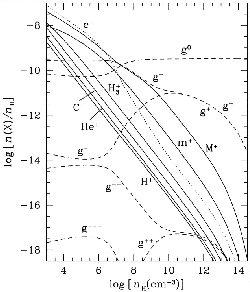In the dense clouds, the ionization fraction is low. Since the uv/optical radiations from stars can not reach the cloud center, potential ionization comes from the cosmic ray particles. In this case the rate of ionization per volume is given as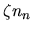, where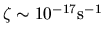. In Figure 4.3, the ionization fraction for various density is shown (Nakano, Nishi, & Umebayashi 2002). This clearly shows that the fraction of ions decrease approximately in proportion to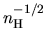for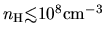. This is understood as follows: Equilibrium balance between one kind of ion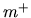and its neutral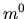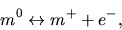(4.35)

is considered. The recombination (reaction from the right to left) rate per unit volume is expressed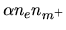, while the ionization rate (left to right) per unit volume is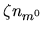, where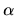means the recombination rate coefficient. Nakano (1984) obtained in the range of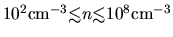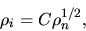(4.36)

where the numerical factor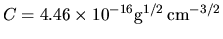.Next: Ambipolar Diffusion Up: Ambipolar Diffusion Previous: Ambipolar Diffusion   Contents
Kohji Tomisaka 2007-07-08